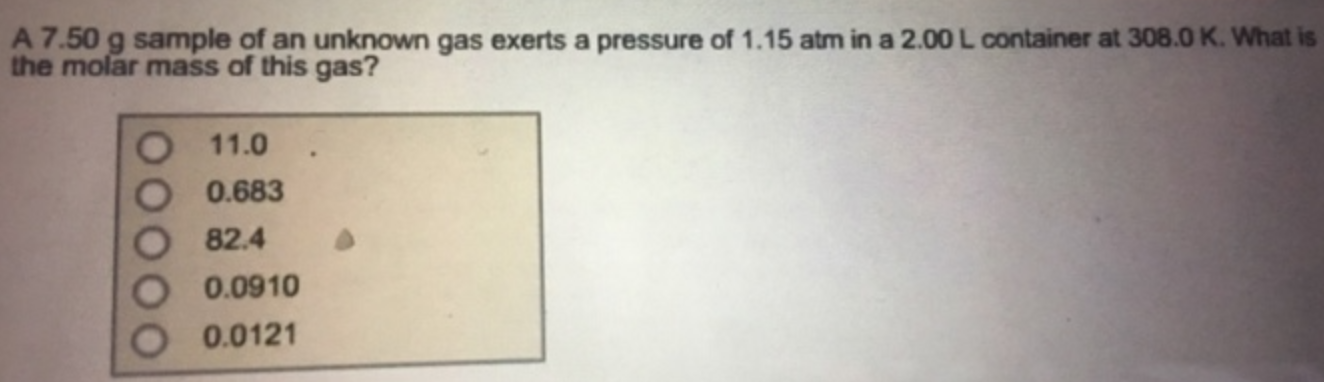Chemistry Practice Problems Ideal Gas Law Molar Mass Practice Problems Solution: A 7.50 g sample of an unknown gas exerts a pressur...

# Solution: A 7.50 g sample of an unknown gas exerts a pressure of 1.15 atm in a 2.00 L container at 306.0 K. What is the molar mass of this gas?

###### Problem

A 7.50 g sample of an unknown gas exerts a pressure of 1.15 atm in a 2.00 L container at 306.0 K. What is the molar mass of this gas?View Complete Written Solution

Ideal Gas Law Molar Mass

Ideal Gas Law Molar Mass

#### Q. A sample of gas has a mass of 827 mg. Its volume is 0.270 L at a temperature of 88°C and a pressure of 975 mmHg. Find its molar mass. Express the mola...

Solved • Tue Jun 19 2018 14:48:22 GMT-0400 (EDT)

Ideal Gas Law Molar Mass

#### Q. What is the volume occupied by 14.0 g of argon gas at a pressure of 1.10 atm and a temperature of 349 K?

Solved • Tue Jun 19 2018 12:50:53 GMT-0400 (EDT)

Ideal Gas Law Molar Mass

#### Q. At STP, 0.278 L of a gas weighs 0.403 g. Calculate the molar mass of the gas. M = _______ g/mol

Solved • Tue Jun 19 2018 11:40:45 GMT-0400 (EDT)

Ideal Gas Law Molar Mass

#### Q. A sample of a gas weighs 2.228 g and has a volume of 810.0 mL at 985.0 torr and 33.0°C. What is its molar mass?

Solved • Tue Jun 05 2018 13:32:46 GMT-0400 (EDT)